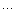# JS中的sleep 、顺序执行

function sleep(msec) {
var k = function_continuation;
setTimeout(function() { resume k <- mesc; }, msec);
suspend;
}

function sleep(numberMillis) {
var now = new Date();
var exitTime = now.getTime() + numberMillis;
while (true) {
now = new Date();
if (now.getTime() > exitTime)
return;
}
}

function sleep(timeout) {
window.showModalDialog("javascript:document.writeln('<script>window.setTimeout(function () { window.close(); }, " + timeout + ");<//script>');");
}

window.alert("before sleep ..."); sleep(2000); window.alert("after sleep ...");

function sleep(millis) {
var notifier = NjsRuntime.createNotifier();
setTimeout(notifier, millis);
notifier.wait->();
}

5
6 Array.prototype.each=function(f){
7     try{
8     for(var i=0;i<this.length;i++){
9         try{
10              f(this[i],i,this)
11         }catch(e){
12             if (e==$breakthrow e 13 } 14 } 15 }catch(e){ 16 } 17 } 18 19 Array.prototype.find=function(f){ 20 var result; 21 this.each(function(value,index,arr){ 22 if (f(value,index,arr)){ 23 result=value 24 throw$break
25         }
26      })
27      return result
28  }
29
30 var arr=[1,2,3,4,5,7,9]
31
32 function foo(v){    //检测是不是偶数
33     return v%2==0
34 }
36
37 </script>prototype.js的代码片段摘取
<!--

Code highlighting produced by Actipro CodeHighlighter (freeware)
http://www.CodeHighlighter.com/

-->var $break = new Object();var$continue = new Object();var Enumerable ={each:
function(iterator){var index = 0;try{this._each(function(value){try{iterator(value, index
++);}
catch (e){if (e != $continuethrow e;}} );} catch (e){if (e !=$breakthrow e;}}
,all:
function(iterator){var result = true;this.each(function(value, index){result
= result && !!(iterator || Prototype.K)(value, index);if (!result) throw $break;} );return result;} ,any: function(iterator){var result = true;this.each(function(value, index){if (result = !!(iterator || Prototype.K)(value, index))throw$break;}
);return result;}
,

### Feedback

Array.prototype.each=function(f){
for(var i=0;i<this.length;i++) if(f(this[i],i,this)==true) break;//,i,this
}

Array.prototype.find=function(){
var result;
this.each(function(value,index,arr){//
//if (f(value,index,arr)) result=value
if (foo(value,index,arr)==true)
{
result=value;
return true;

}
})
return result;
}

var arr=[1,2,3,4,5,7,9];

function foo(v){ //检测是不是偶数
if(v%2==0)return true;
}